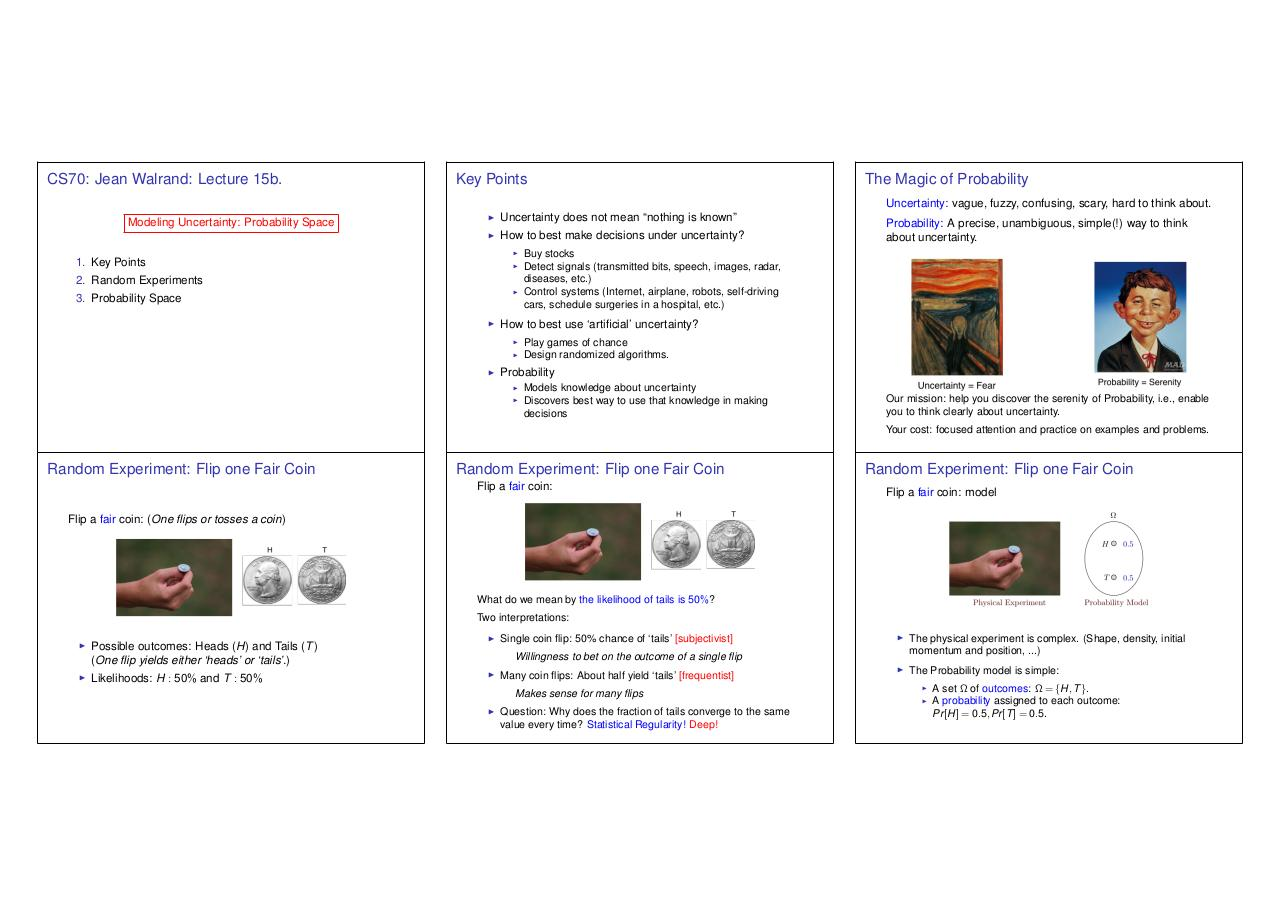# lec 15.6up.pdfPage 1 2 3 4 5 6 7

#### Text preview

CS70: Jean Walrand: Lecture 15b.

Key Points

The Magic of Probability
Uncertainty: vague, fuzzy, confusing, scary, hard to think about.

Modeling Uncertainty: Probability Space

I

Uncertainty does not mean “nothing is known”

I

How to best make decisions under uncertainty?
I

1. Key Points

I

2. Random Experiments

I

3. Probability Space
I

Detect signals (transmitted bits, speech, images, radar,
diseases, etc.)
Control systems (Internet, airplane, robots, self-driving
cars, schedule surgeries in a hospital, etc.)

How to best use ‘artificial’ uncertainty?
I
I

I

Probability: A precise, unambiguous, simple(!) way to think

Play games of chance
Design randomized algorithms.

Probability
I
I

Discovers best way to use that knowledge in making
decisions

you to think clearly about uncertainty.
Your cost: focused attention and practice on examples and problems.

Random Experiment: Flip one Fair Coin

Random Experiment: Flip one Fair Coin
Flip a fair coin:

Random Experiment: Flip one Fair Coin
Flip a fair coin: model

Flip a fair coin: (One flips or tosses a coin)

What do we mean by the likelihood of tails is 50%?
Two interpretations:
I

Possible outcomes: Heads (H) and Tails (T )
(One flip yields either ‘heads’ or ‘tails’.)

I

Likelihoods: H : 50% and T : 50%

I

Single coin flip: 50% chance of ‘tails’ [subjectivist]

I

The physical experiment is complex. (Shape, density, initial
momentum and position, ...)

I

The Probability model is simple:

Willingness to bet on the outcome of a single flip
I

Many coin flips: About half yield ‘tails’ [frequentist]
Makes sense for many flips

I

Question: Why does the fraction of tails converge to the same
value every time? Statistical Regularity! Deep!

I
I

A set ⌦ of outcomes: ⌦ = {H, T }.
A probability assigned to each outcome:
Pr [H] = 0.5, Pr [T ] = 0.5.